11. Generalized Exchange and Laws of Conservation

L. G. Kreidik (translation from Russian T. S. Kortneva and G. P. Shpenkov)

We will consider kinematic exchange between a system and the environment

on the Z-level of rest-motion (Fig. 2.15).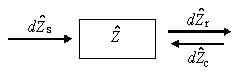Fig. 2.15. A graph of Z-level exchange.

Let motion-rest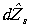be transferred from the environment to the system and the amount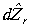of motion-rest be transferred from the system to the environment along the kinetic channel and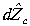be transferred by the system over the potential. If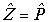, then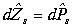,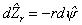,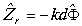, (2.262)

where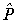is a parameter of any level of motion, r is kinetic resistance or kinetic elasticity, k is potential resistance or potential elasticity,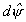and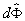are differentials of particular states.

In a general case, the resistances of the exchange channels depend on the state of the system, environment, and the character of the exchange channels; in the linear approximation they are constant. Their inverse values, g and C , will be called kinetic and potential conductivities, respectively.

Each of the differentials of exchange over a direct and two inverse channels determines the amount of mutual exchange equal to the difference of partial components of exchange. The rest-motion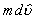gained by the system is equal to the sum of exchanges in the three channels. Thus, we have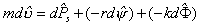. (2.263)

Hence, we arrive at the equation of exchange in the form: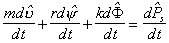(2.264)

or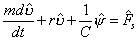(2.264a)

or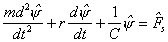. (2.264b)

The equation of exchange is simultaneously the equation of the state of the system.

We will write the exchange-state equations for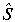-,-,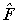- and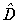- levels: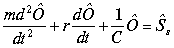,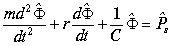, (2.265)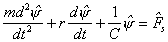,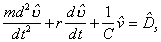(2.266)

or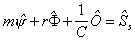,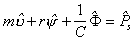, (2.267)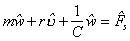,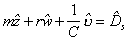. (2.268)

In a broad sense, the first terms in the left-hand sides of the equations are kinetic momenta, the second and third terms are kinetic and potential momenta of the feedback with the environment.

If we introduce the generalized charge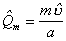,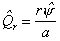,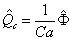, (2.269)

where a is the characteristic length, then in terms of charges the equation for the-level becomes: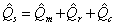. (2.270)

For the-level it will be represented by the equation of current: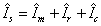. (2.271)

Finally, on the-level the equation takes the form: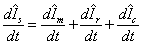. (2.272)

If the system is closed over the channel(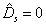), it is closed over all overlying channels and in a general case, it is not closed over all underlying channels

Energy description of the levels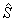,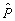,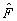and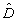is expressed by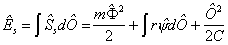, (2.273)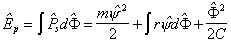, (2.274)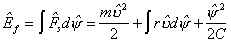, (2.275)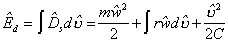. (2.276)

If the system is closed over the kinetic channel, i.e.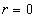, then energies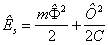,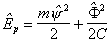, (2.277)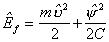,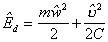, (2.278)

are conserved. If the system is open, motion-rest is also conserved but within the common bounds of the system and environment.

Theoretical Dialectical Journal: Physics-Mathematics-Logic-Philosophy, N.2, site http://www.tedial.narod.ru/